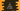# 5 different ways to find the volume of a cube in C## Introduction :

In this tutorial, we will learn how to find the volume of a cube in C programming language. The program will take the side of the cube as input and prints the volume. The formula used to calculate the volume of a cube is as below :

``Volume = side ^ 3``

If the side is 2 cm, its volume will be 8 cm^3.

I will show you different ways to solve this problem. Go through the examples and drop one comment below if you have any questions.

### Method 1: Standard way :

Let’s take a look at the below program :

``````#include <stdio.h>

int main()
{
float side, volume;

side = 4;
volume = side * side * side;

printf("The volume is : %.02f\n", volume);
return 0;
}``````

Here, we are using two floating-point variables side and volume. This program calculates the volume for side = 4.

We are multiplying the value of side three times to get the cube of it.

It will print the below output :

``The volume is : 64.00``

### Method 2: Using math.pow() function :

math.h header library defines one function called pow() to find out the power of a number. We can use it to find the cube of a number as like below :

``````#include <stdio.h>
#include <math.h>

int main()
{
float side, volume;

side = 4;
volume = pow(side, 3);

printf("The volume is : %.02f\n", volume);
return 0;
}``````

It will print :

``The volume is : 64.00``

### Method 3: By taking the side as user input :

We can use scanf to read the side as user input and calculate the volume as like below :

``````#include <stdio.h>
#include <math.h>

int main()
{
float side, volume;

printf("Enter the side of the cube : ");
scanf("%f",&side);

volume = pow(side, 3);

printf("The volume is : %.02f\n", volume);
return 0;
}``````

It reads the side into the variable side and calculates the volume.

It can take any value as side and calculate the value of volume. One sample output :

``````Enter the side of the cube : 6
The volume is : 216.00

Enter the side of the cube : 4
The volume is : 64.00``````

### Method 4: Using a different function :

We can use one different function to do the calculation and the main function can call this function :

``````#include <stdio.h>
#include <math.h>

float findCube(float side)
{
return pow(side, 3);
}
int main()
{
float side, volume;

printf("Enter the side of the cube : ");
scanf("%f", &side);

volume = findCube(side);

printf("The volume is : %.02f\n", volume);
return 0;
}``````

Using a different function is useful if you want to reuse that function. We can put the implementation in one place and call that method from other places.

### Method 5: Using pointers :

We can also use pointers to do the same thing as below :

``````#include <stdio.h>
#include <math.h>

float findCube(float *side)
{
return pow(*side, 3);
}
int main()
{
float side, volume;

printf("Enter the side of the cube : ");
scanf("%f", &side);

volume = findCube(&side);

printf("The volume is : %.02f\n", volume);
return 0;
}``````

Here, we are passing the address of side to findCube. *side gets the value of side.